# Python | Pandas tseries.offsets.DateOffset.rule_code

Dateoffsets are a standard kind of date increment used for a date range in Pandas. It works exactly like relativedelta in terms of the keyword args we pass in. DateOffets work as follows, each offset specify a set of dates that conform to the DateOffset. For example, Bday defines this set to be the set of dates that are weekdays (M-F).

DateOffsets can be created to move dates forward a given number of valid dates. For example, Bday(2) can be added to a date to move it two business days forward. If the date does not start on a valid date, first it is moved to a valid date and then offset is created.

Pandas` tseries.offsets.DateOffset.rule_code` attribute returns the rule_code applied on the given DateOffset object. For example the rule_code of BMonthEnd is ‘BM’.

Syntax:
pandas.tseries.offsets.DateOffset.rule_code

Parameter : None

Returns : rule_code

Example #1: Use `pandas.tseries.offsets.DateOffset.rule_code` attribute to return the rule_code applied on the given DateOffset object.

 `# importing pandas as pd ` `import` `pandas as pd ` ` `  `# importing the to_offset function ` `from` `pandas.tseries.frequencies ``import` `to_offset ` ` `  `# Creating Timestamp ` `ts ``=` `pd.Timestamp(``'2019-10-10 07:15:11'``) ` ` `  `# Create the DateOffset of 2 day ` `do ``=` `to_offset(freq ``=` `'2D'``) ` ` `  `# Print the Timestamp ` `print``(ts) ` ` `  `# Print the DateOffset ` `print``(do) `

Output :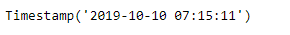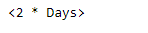Now we will add the dateoffset to the given timestamp object to increment the datetime value. We will also return the rule_code applied on the given DateOffset object.

 `# Adding the dateoffset to the given timestamp ` `new_timestamp ``=` `ts ``+` `do ` ` `  `# Print the updated timestamp ` `print``(new_timestamp) ` ` `  `# Now we will print the rule_code applied ` `# on the given DateOffset object ` `print``(do.rule_code) `

Output :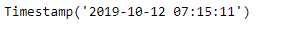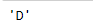As we can see in the output, the attribute has successfully returned the rule_code applied on the given Dateoffset object.

Example #2: Use `pandas.tseries.offsets.DateOffset.rule_code` attribute to return the rule_code applied on the given DateOffset object.

 `# importing pandas as pd ` `import` `pandas as pd ` ` `  `# importing the to_offset function ` `from` `pandas.tseries.frequencies ``import` `to_offset ` ` `  `# Creating Timestamp ` `ts ``=` `pd.Timestamp(``'2019-10-10 07:15:11'``) ` ` `  `# Create the DateOffset ` `do ``=` `to_offset(freq ``=` `'10D2H'``) ` ` `  `# Print the Timestamp ` `print``(ts) ` ` `  `# Print the DateOffset ` `print``(do) `

Output :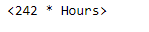Now we will add the dateoffset to the given timestamp object to increment the datetime value. We will also return the rule_code applied on the given DateOffset object.

 `# Adding the dateoffset to the given timestamp ` `new_timestamp ``=` `ts ``+` `do ` ` `  `# Print the updated timestamp ` `print``(new_timestamp) ` ` `  `# Now we will print the rule_code applied ` `# on the given DateOffset object ` `print``(do.rule_code) `

Output :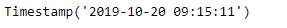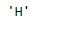As we can see in the output, the attribute has successfully returned the rule_code applied on the given Dateoffset object.

My Personal Notes arrow_drop_upCheck out this Author's contributed articles.

If you like GeeksforGeeks and would like to contribute, you can also write an article using contribute.geeksforgeeks.org or mail your article to contribute@geeksforgeeks.org. See your article appearing on the GeeksforGeeks main page and help other Geeks.

Please Improve this article if you find anything incorrect by clicking on the "Improve Article" button below.

Article Tags :

Be the First to upvote.

Please write to us at contribute@geeksforgeeks.org to report any issue with the above content.